Algebra Commons™

1,047 Full-Text Articles 1,224 Authors 224,226 Downloads118 Institutions

All Articles in Algebra

1,047 full-text articles. Page 7 of 39.

Preface: International Conference On Matrix Analysis And Its Applications -- Mattriad 2017, 2018University of Tampere

Preface: International Conference On Matrix Analysis And Its Applications -- Mattriad 2017, Oskar Maria Baksalary, Natalia Bebiano, Heike Fassbender, Simo Puntanen

Electronic Journal of Linear Algebra

No abstract provided.

Norm Inequalities Related To Clarkson Inequalities, 2018University of Jordan

Norm Inequalities Related To Clarkson Inequalities, Fadi Alrimawi, Omar Hirzallah, Fuad Kittaneh

Let $A$ and $B$ be $n\times n$ matrices. It is shown that if $p=2$, $4\leq p<\infty$, or $2 Bounds For The Completely Positive Rank Of A Symmetric Matrix Over A Tropical Semiring, 2018University of Ljubljana Bounds For The Completely Positive Rank Of A Symmetric Matrix Over A Tropical Semiring, David Dolžan, Polona Oblak Electronic Journal of Linear Algebra In this paper, an upper bound for the CP-rank of a matrix over a tropical semiring is obtained, according to the vertex clique cover of the graph prescribed by the positions of zero entries in the matrix. The graphs that beget the matrices with the lowest possible CP-ranks are studied, and it is proved that any such graph must have its diameter equal to$2$. 2018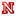University of Nebraska-Lincoln Resolutions Of Finite Length Modules Over Complete Intersections, Seth Lindokken Dissertations, Theses, and Student Research Papers in Mathematics The structure of free resolutions of finite length modules over regular local rings has long been a topic of interest in commutative algebra. Conjectures by Buchsbaum-Eisenbud-Horrocks and Avramov-Buchweitz predict that in this setting the minimal free resolution of the residue field should give, in some sense, the smallest possible free resolution of a finite length module. Results of Tate and Shamash describing the minimal free resolution of the residue field over a local hypersurface ring, together with the theory of matrix factorizations developed by Eisenbud and Eisenbud-Peeva, suggest analogous lower bounds for the size of free resolutions of finite length ... 2018University of Nebraska - Lincoln Supporting English Language Learners Inside The Mathematics Classroom: One Teacher’S Unique Perspective Working With Students During Their First Years In America, Amy Marie Fendrick Research and Evaluation in Literacy and Technology Reflecting upon my personal experiences teaching mathematics to English Language Learners (ELL) in a public high school in Lincoln, Nebraska, this essay largely focuses on the time I spent as the only Accelerated Math teacher in my school building. From 2012 – 2017, I taught three different subjects at this high school: Advanced Algebra, Algebra, and Accelerated Math. This essay highlights why I chose to become a math and ELL teacher, as well as the challenges, issues, struggles, and successes I experienced during my time teaching. I focus on the challenges I faced teaching students who did not share my native ... Tp Matrices And Tp Completability, 2018William & Mary Tp Matrices And Tp Completability, Duo Wang Undergraduate Honors Theses A matrix is called totally nonnegative (TN) if the determinant of every square submatrix is nonnegative and totally positive (TP) if the determinant of every square submatrix is positive. The TP (TN) completion problem asks which partial matrices have a TP (TN) completion. In this paper, several new TP-completable pat- terns in 3-by-n matrices are identied. The relationship between expansion and completability is developed based on the prior re- sults about single unspecied entry. These results extend our un- derstanding of TP-completable patterns. A new Ratio Theorem related to TP-completability is introduced in this paper, and it can possibly be ... 2018College of William and Mary Strongly Real Conjugacy Classes In Unitary Groups Over Fields Of Even Characteristic, Tanner N. Carawan Undergraduate Honors Theses An element$g$of a group$G$is called strongly real if there is an$s$in$G$such that$s^2 = 1$and$sgs^{-1} = g^{-1}$. It is a fact that if$g$in$G$is strongly real, then every element in its conjugacy class is strongly real. Thus we can classify each conjugacy class as strongly real or not strongly real. Gates, Singh, and Vinroot have classified the strongly real conjugacy classes of U$(n, q^2)$in the case that$q$is odd. Vinroot and Schaeffer Fry have classified some of the conjugacy classes of U ... 2018William & Mary Counting Real Conjugacy Classes In Some Finite Classical Groups, Elena Amparo Undergraduate Honors Theses An element$g$in a group$G$is real if there exists$x\in G$such that$xgx^{-1}=g^{-1}$. If$g$is real then all elements in the conjugacy class of$g$are real. In \cite{GS1} and \cite{GS2}, Gill and Singh showed that the number of real$\mathrm{GL}_n(q)$-conjugacy classes contained in$\mathrm{SL}_n(q)$equals the number of real$\mathrm{PGL}_n(q)$-conjugacy classes when$q$is even or$n$is odd. In this paper, we use generating functions to show that the result is also true for odd$q ...

The Doubly Stochastic Single Eigenvalue Problem: An Empirical Approach, 2018College of William & Mary

The Doubly Stochastic Single Eigenvalue Problem: An Empirical Approach, John Wilkes, Charles Royal Johnson

The doubly stochastic single eigenvalue problem asks what is the set DSn of all complex numbers that occur as an eigenvalue of an n-by-n doubly stochastic matrix. For n < 5, this set is known and for the analogous set for (singly) stochastic matrices, the set is known for all n. For Pk , the polygon formed by the k-th roots of unity, Unk=1 Pk ⊆ DSn, as is easily shown. For n < 5, this containment is an equality, but for n = 5, the containment is strict (though it is close). Presented here is substantial, computational evidence that the containment is an equality for 6 ≤ n ≤ 10 and for what DS5 actually is.

2018William & Mary

A New Upper Bound For The Diameter Of The Cayley Graph Of A Symmetric Group, Hangwei Zhuang

Given a finite symmetric group S_n and a set S of generators, we can represent the group as a Cayley graph. The diameter of the Cayley graph is the largest distance from the identity to any other elements. We work on the conjecture that the diameter of the Cayley graph of a finite symmetric group S_n with S ={(12),(12...n)} is at most $C(n,2). Our main result is to show that the diameter of the graph of S_n is at most (3n^2-4n)/2. Nonassociative Right Hoops, 2018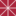Chapman University Nonassociative Right Hoops, Peter Jipsen, Michael Kinyon Mathematics, Physics, and Computer Science Faculty Articles and Research The class of nonassociative right hoops, or narhoops for short, is defined as a subclass of right-residuated magmas, and is shown to be a variety. These algebras generalize both right quasigroups and right hoops, and we characterize the subvarieties in which the operation x ^^ y = (x/y)y is associative and/or commutative. Narhoops with a left unit are proved to be integral if and only if ^ is commutative, and their congruences are determined by the equivalence class of the left unit. We also prove that the four identities defining narhoops are independent. 2018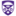The University of Western Ontario Putting Fürer's Algorithm Into Practice With The Bpas Library, Linxiao Wang Electronic Thesis and Dissertation Repository Fast algorithms for integer and polynomial multiplication play an important role in scientific computing as well as other disciplines. In 1971, Schönhage and Strassen designed an algorithm that improved the multiplication time for two integers of at most n bits to O(log n log log n). In 2007, Martin Fürer presented a new algorithm that runs in O (n log n · 2 ^O(log* n)) , where log*n is the iterated logarithm of n. We explain how we can put Fürer’s ideas into practice for multiplying polynomials over a prime field Z/pZ, which characteristic is a Generalized ... Upper Bound For The Number Of Distinct Eigenvalues Of A Perturbed Matrix, 2018Sookmyung Women's University, Upper Bound For The Number Of Distinct Eigenvalues Of A Perturbed Matrix, Sunyo Moon, Seungkook Park Electronic Journal of Linear Algebra In 2016, Farrell presented an upper bound for the number of distinct eigenvalues of a perturbed matrix. Xu (2017), and Wang and Wu (2016) introduced upper bounds which are sharper than Farrell's bound. In this paper, the upper bounds given by Xu, and Wang and Wu are improved. Range-Compatible Homomorphisms Over The Field With Two Elements, 2018Université de Versailles Saint-Quentin-en-Yvelines, Laboratoire de Mathématiques de Versailles Range-Compatible Homomorphisms Over The Field With Two Elements, Clément De Seguins Pazzis Electronic Journal of Linear Algebra Let U and V be finite-dimensional vector spaces over a field K, and S be a linear subspace of the space L(U, V ) of all linear operators from U to V. A map F : S → V is called range-compatible when F(s) ∈ Im s for all s ∈ S. Previous work has classified all the range-compatible group homomorphisms provided that codimL(U,V )S ≤ 2 dim V − 3, except in the special case when K has only two elements and codimL(U,V )S = 2 dim V − 3. This article gives a thorough treatment of that special case. The results ... 2018William & Mary Implementation And Analysis Of The Nonlinear Decomposition Attack On Polycyclic Groups, Yoongbok Lee Undergraduate Honors Theses Around two years ago, Roman'kov introduced a new type of attack called the nonlinear decomposition attack on groups with solvable membership search problem. To analyze the precise efficiency of the algorithm, we implemented the algorithm on two protocols: semidirect product protocol and Ko-Lee protocol. Because polycyclic groups were suggested as possible platform groups in the semidirect product protocol and polycyclic groups have a solvable membership search problem, we used poly- cyclic groups as the platform group to test the attack. While the complexity could vary regarding many different factors within the group, there was always at least one exponential ... 2018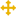John Carroll University An Investigation Into The Properties Of Quaternions: Their Origin, Basic Properties, Functional Analysis, And Algebraic Characteristics, James Miller Masters Essays No abstract provided. Linear Algebra (Ung), 2018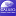University of North Georgia Linear Algebra (Ung), Hashim Saber, Beata Hebda, Piotr Hebda, Benkam Bobga Mathematics Grants Collections This Grants Collection for Linear Algebra was created under a Round Seven ALG Textbook Transformation Grant. Affordable Learning Georgia Grants Collections are intended to provide faculty with the frameworks to quickly implement or revise the same materials as a Textbook Transformation Grants team, along with the aims and lessons learned from project teams during the implementation process. Documents are in .pdf format, with a separate .docx (Word) version available for download. Each collection contains the following materials: • Linked Syllabus • Initial Proposal • Final Report Cayley Graphs Of Psl(2) Over Finite Commutative Rings, 2018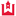Western Kentucky University Cayley Graphs Of Psl(2) Over Finite Commutative Rings, Kathleen Bell Masters Theses & Specialist Projects Hadwiger's conjecture is one of the deepest open questions in graph theory, and Cayley graphs are an applicable and useful subtopic of algebra. Chapter 1 will introduce Hadwiger's conjecture and Cayley graphs, providing a summary of background information on those topics, and continuing by introducing our problem. Chapter 2 will provide necessary definitions. Chapter 3 will give a brief survey of background information and of the existing literature on Hadwiger's conjecture, Hamiltonicity, and the isoperimetric number; in this chapter we will explore what cases are already shown and what the most recent results are. Chapter 4 will ... Potential Stability Of Matrix Sign Patterns, 2018William & Mary Potential Stability Of Matrix Sign Patterns, Christopher Hambric Undergraduate Honors Theses The topic of matrix stability is very important for determining the stability of solutions to systems of differential equations. We examine several problems in the field of matrix stability, including minimal conditions for a$7\times7$matrix sign pattern to be potentially stable, and applications of sign patterns to the study of Turing instability in the$3\times3\$ case. Furthermore, some of our work serves as a model for a new method of approaching similar problems in the future.

2018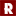Rose-Hulman Institute of Technology

Branching Matrices For The Automorphism Group Lattice Of A Riemann Surface, Sean A. Broughton

Mathematical Sciences Technical Reports (MSTR)

Let S be a Riemann surface and G a large subgroup of Aut(S) (Aut(S) may be unknown). We are particularly interested in regular n-gonal surfaces, i.e., the quotient surface S/G (and hence S/Aut(S)) has genus zero. For various H the ramification information of the branched coverings S/K -> S/H may be captured in a matrix. The ramification information, in particular strong branching, may be then be used in analyzing the structure of Aut(S). The ramification information is conjugation invariant so the matrix's rows and columns may be indexed by conjugacy ...